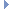OpenMM
AmoebaInPlaneAngleForce Class Reference

This class implements an interaction at trigonal centers corresponding to the projected in-plane angle bend energy between four particles. More...Inheritance diagram for AmoebaInPlaneAngleForce:

List of all members.

## Public Member Functions

def getNumAngles
getNumAngles(self) -> int
def setAmoebaGlobalInPlaneAngleCubic
Set the global cubic term.
def getAmoebaGlobalInPlaneAngleCubic
getAmoebaGlobalInPlaneAngleCubic(self) -> double
def setAmoebaGlobalInPlaneAngleQuartic
Set the global quartic term.
def getAmoebaGlobalInPlaneAngleQuartic
getAmoebaGlobalInPlaneAngleQuartic(self) -> double
def setAmoebaGlobalInPlaneAnglePentic
Set the global pentic term.
def getAmoebaGlobalInPlaneAnglePentic
getAmoebaGlobalInPlaneAnglePentic(self) -> double
def setAmoebaGlobalInPlaneAngleSextic
Set the global sextic term.
def getAmoebaGlobalInPlaneAngleSextic
getAmoebaGlobalInPlaneAngleSextic(self) -> double
def getAngleParameters
Get the force field parameters for an angle term.
def setAngleParameters
Set the force field parameters for an angle term.
def updateParametersInContext
Update the per-angle parameters in a Context to match those stored in this Force object.
def usesPeriodicBoundaryConditions
usesPeriodicBoundaryConditions(self) -> bool
def __init__
__init__(self) -> AmoebaInPlaneAngleForce __init__(self, other) -> AmoebaInPlaneAngleForce

this

## Detailed Description

This class implements an interaction at trigonal centers corresponding to the projected in-plane angle bend energy between four particles.

The interaction is defined by a 6th order polynomial in the angle between them. Only the quadratic term is set per-angle. The coefficients of the higher order terms each have a single value that is set globally.

To use it, create an AmoebaInPlaneAngleForce object then call addAngle() once for each angle. After an angle has been added, you can modify its force field parameters by calling setAngleParameters(). This will have no effect on Contexts that already exist unless you call updateParametersInContext().

## Constructor & Destructor Documentation

 def __init__ ( self, args )

__init__(self) -> AmoebaInPlaneAngleForce __init__(self, other) -> AmoebaInPlaneAngleForce

Create an AmoebaAngleForce.

## Member Function Documentation

Add an angle term to the force field.

Parameters:
 particle1 (int) the index of the first particle connected by the angle particle2 (int) the index of the second particle connected by the angle particle3 (int) the index of the third particle connected by the angle particle4 (int) the index of the fourth particle connected by the angle length (double) the equilibrium angle, measured in radians quadraticK (double) the quadratic force constant for the angle measured in kJ/mol/radian^2
Returns:
(int) the index of the angle that was added
 def getAmoebaGlobalInPlaneAngleCubic ( self )

getAmoebaGlobalInPlaneAngleCubic(self) -> double

Get the global cubic term

Returns:
(double) global cubicK term
 def getAmoebaGlobalInPlaneAnglePentic ( self )

getAmoebaGlobalInPlaneAnglePentic(self) -> double

Get the global pentic term

Returns:
(double) global penticK term
 def getAmoebaGlobalInPlaneAngleQuartic ( self )

getAmoebaGlobalInPlaneAngleQuartic(self) -> double

Get the global quartic term

Returns:
(double) global quartic term
 def getAmoebaGlobalInPlaneAngleSextic ( self )

getAmoebaGlobalInPlaneAngleSextic(self) -> double

Get the global sextic term

Returns:
(double) global sextic term
 def getAngleParameters ( self, index )

Get the force field parameters for an angle term.

Parameters:
 index (int) the index of the angle for which to get parameters
Returns:
(int) the index of the first particle connected by the angle
(int) the index of the second particle connected by the angle
(int) the index of the third particle connected by the angle
(int) the index of the fourth particle connected by the angle
(double) the equilibrium angle, measured in radians
 def getNumAngles ( self )

getNumAngles(self) -> int

Get the number of in-plane angle terms in the potential function

 def setAmoebaGlobalInPlaneAngleCubic ( self, cubicK )

Set the global cubic term.

Parameters:
 cubicK (double) the cubic force constant for the angle
 def setAmoebaGlobalInPlaneAnglePentic ( self, penticK )

Set the global pentic term.

Parameters:
 penticK (double) the pentic force constant for the angle
 def setAmoebaGlobalInPlaneAngleQuartic ( self, quarticK )

Set the global quartic term.

Parameters:
 quarticK (double) the quartic force constant for the angle
 def setAmoebaGlobalInPlaneAngleSextic ( self, sexticK )

Set the global sextic term.

Parameters:
 sexticK (double) the sextic force constant for the angle
 def setAngleParameters ( self, index, particle1, particle2, particle3, particle4, length, quadraticK )

Set the force field parameters for an angle term.

Parameters:
 index (int) the index of the angle for which to set parameters particle1 (int) the index of the first particle connected by the angle particle2 (int) the index of the second particle connected by the angle particle3 (int) the index of the third particle connected by the angle particle4 (int) the index of the fourth particle connected by the angle length (double) the equilibrium angle, measured in radians quadraticK (double) the quadratic force constant for the angle, measured in kJ/mol/radian^2
 def updateParametersInContext ( self, context )

Update the per-angle parameters in a Context to match those stored in this Force object.

This method provides an efficient method to update certain parameters in an existing Context without needing to reinitialize it. Simply call setAngleParameters() to modify this object's parameters, then call updateParametersInContext() to copy them over to the Context.

The only information this method updates is the values of per-angle parameters. The set of particles involved in an angle cannot be changed, nor can new angles be added.

 def usesPeriodicBoundaryConditions ( self )

usesPeriodicBoundaryConditions(self) -> bool

Returns whether or not this force makes use of periodic boundary conditions.

Returns:
(bool) true if nonbondedMethod uses PBC and false otherwise

Reimplemented from Force.

## Member Data Documentation

Reimplemented from Force.

The documentation for this class was generated from the following file:
• openmm.py